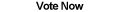Home Articles Components Code Snippets Links

C# Code Snippets# C# Factorial

Visual C# Kicks

The C# factorial function uses long variables since factorial values get large very quickly. The lowerbound parameter makes dividing factorials possible since the entire value usually does not need to be calculated.

Platform: .NET Framework 2.0

```public long Factorial(long x)
{
long fact = 1;
long i = 1;
while (i <= x)
{
fact = fact * i;
i++;
}
return fact;
}

public long Factorial(long x, long lowerBound)
{
long fact = 1;
while (x >= 1 && x > lowerBound)
{
fact *= x;
x--;
}

return fact;
}```

Back to C# Code Snippet List